# Math / Science

9K

Solutions

6K

Contributors

The Math / Science topic primarily includes discussions of mathematics, physics, statistics and economic analysis, but also biology, chemistry and other sciences.

Can someone please explain to me what process to go through to find the steepest point on a curve, I’ve seen other people say to find the max and min but I thought those were the points where the slope was flat?? This is for my assignment and Im pretty stuck
0
1.      One country has license plates for cars that have 3 letters followed by 3 digits, or 3 digits followed by 3 letters. A second country has license plates with four letters followed by three digits. Assuming that zero can be the first digit in a three-digit license number, how many more license plates can be made using a 4-letter, 3- digit format than can be made with a 3-letter, 3- digit format?

2.      School calculators are sold in packages of 11 and packages of 15. What is the largest number of calculators it is not possible to purchase without opening a package?

3.      Sixteen students have registered for a summer sports camp. They are to be divided into either a swimming or a tennis activity, with no more than 10 students in either group. In how many ways could the group in the tennis activity be chosen?
0
I calculate it using the formula but I'm not sure if its right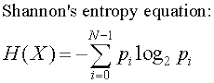(a) The random variable X that takes the values 0, 1, 2 with probability 1/3.

(b) The random variable X that takes the values 1, 2, 3, 4 with probability 1/4.

(c) The random variable X that takes the values in {1, . . . , 1000} uniformly at random.

(d) The random variable X that takes the values in {0, . . . , 1023} uniformly at random.
0
Hi everyone,

in SAS I am trying to sort one SAS dataset by a certain variable (var1) and then write all records to SAS datasets (name includes "var1") and ASCII files (name includes "var1"). I am thinking to do everything in two steps as below but I am not sure if Step 2 should be done in PROC SQL or maybe in a DATA step with macros?  If anyone knows how to do Step 2 please let me know. Any suggestions or examples would be greatly appriciated.

Step 1. Sort olddata.sas7bdat SAS dataset by var1
Step 2. Write all records from olddata.sas7bdat for each unique var1 to its correcponding data<var1>.sas7bdat SAS datasets and data<var1>.in ASCII files

``````*********************
* Step 1- sorting initial dataset
***********************
proc sort data=olddata;
by var1;
run;

****************************
* Step 2 - creating data<var1>.sas7bdat
****************************
proc sql noprint;
select distinct var1 into : x separated by ' ' from olddata;
quit;
%macro create;
%do i = 1 %to %eval(%sysfunc(count(&x, %str( )))+1);
data data%scan(&x,&i.);
set olddata;
if var1 = "%scan(&x,&i.)";
run;
%end;
%mend;
%create
``````
0
Hello,
I get the number of minutes from a database.
The number of minutes should be displayed as a line in a coordinate system.
For a minute, I take a pixel.
But if I want to display 20 hours, then the line is too long.
Is there a way to display the minutes exactly by scaling down or up (without having to round)?

Is it possible to display for a minute 0.5 pixel?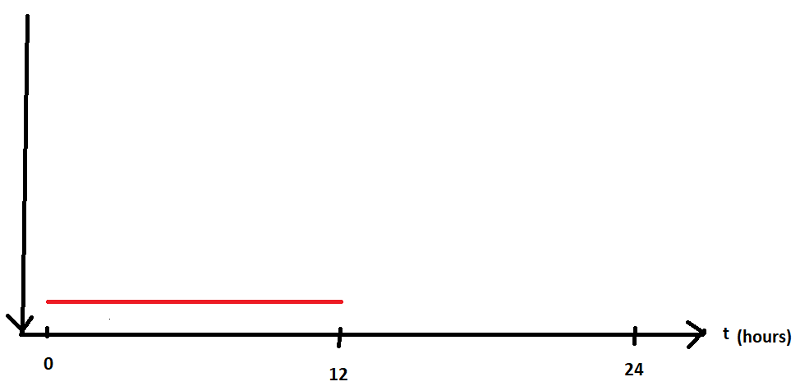Thank you
0
Hello,
I have on my program several rectangles. The rectangles are moveable.  But Collisions should be avoided when moving.
For example:
When I move the blue rectangle on the red rectangle then a collision happened.
The blue rectangle should then be placed under the red rectangle.
But then a collision with the green rectangle happens.
So rectangle blue should be placed between rectangle green and red.
I draw a rectangle after mouseUp Event.

How can I solve this problem?Result: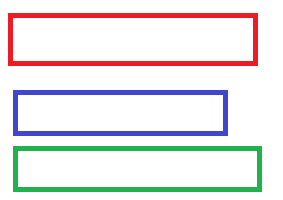Thank you
0
Dear experts,
I am preparing for Financial Engineering.
I recently came across one particular discussion that Game Theory and Graph theory from Batchelor’s level mathematics is required for Financial Engineering.

I am currently taking guidance from a Phd Student. He went through the below books and did not come across these two topics- Game Theory and Graph theory

1. Solutions Manual - A Linear Algebra Primer for Financial Engineering... \$40.00
Qty: 1
Sold By: Amazon.com Services, Inc.

2. A Linear Algebra Primer for Financial Engineering: Covariance... \$62.00
Qty: 1
Sold By: Amazon.com Services, Inc.

3. Solutions Manual - A Primer For The Mathematics Of Financial... \$40.00
Qty: 1
Sold By: Amazon.com Services, Inc.

4. A Primer For The Mathematics Of Financial Engineering, Second Edition... \$54.43

Stochastic Calculus by Steven Shreve: book 1
https://www.amazon.com/Stochastic-Calculus-Finance-Binomial-Springer/dp/0387249680/ref=sr_1_1?crid=YCDXWOSM5YBB&keywords=stochastic+calculus+for+finance+i&qid=1553153578&s=gateway&sprefix=stochastic+calculus%2Caps%2C676&sr=8-1

Stochastic Calculus by Steven Shreve: book 2
https://www.amazon.com/Stochastic-Calculus-Finance-II-Continuous-Time/dp/144192311X/ref=sr_1_1?keywords=steven+shreve&qid=1553153123&s=gateway&sr=8-1

Can someone kindly guide me if these two topics (Game Theory and Graph theory) are relevant for financial engineering.

Thank you
0
I'm trying to figure out an algorithm for the following problem:

- There should always be two rectangles connected to a line
- The lines always run from the middle of the rectangle1 to the center of the rectangle2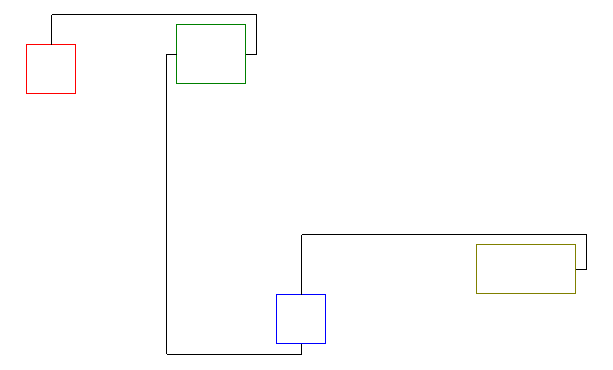- One rectangle has two inputs and two outputs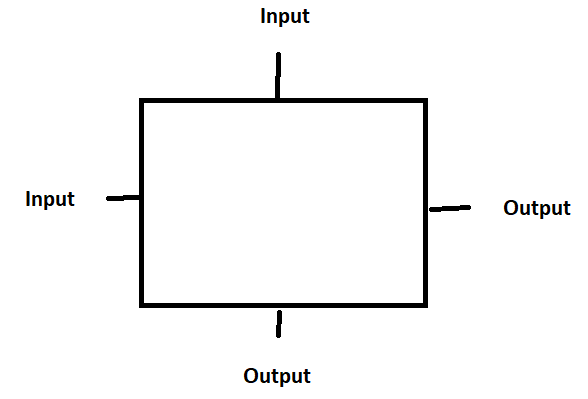- An output can only be connected to one input
- An input can only be connected to one output
- The first rectangle has a connection from the output to the input
- You can move the rectangles as you like
- All lines must be outside the rectangles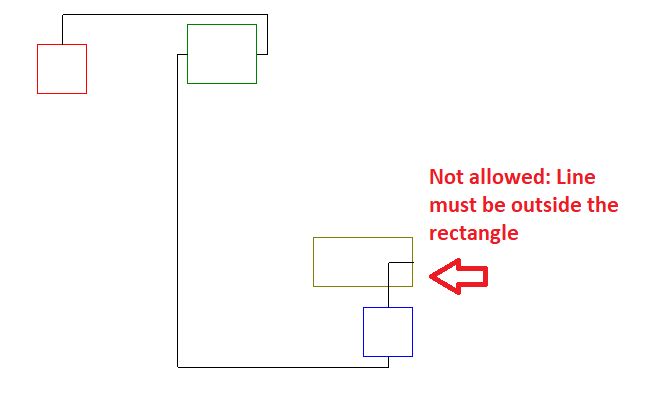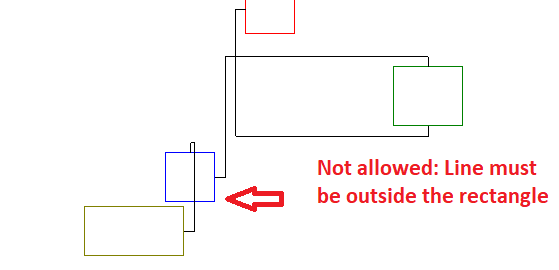- The lines may intersect

My idea is to always compare two rectangles. The left and the right rectangle.
I first determine the left rectangle of the two rectangles.
Then I ask if the left rectangle is above or below the right rectangle and draw the lines accordingly

In addition, I still have to query whether the center of the smaller rectangle is within the larger rectangle and draw the lines, then different.

My questions:
1) Is this algorithm feasible under these conditions?
2) What else do I have to keep in mind about my algorithm, or do you have ideas for a better algorithm?

I am very grateful for numerous tips
0
Hi,
I'm using Excel Solver to run regressions on 63 factors and getting below par results.
Can anyone advise how I can beef up the resultant coefficients. ?
How can I determine factors that have a correlation ?
One problem which I have no power over is that some of the ranked variables that I am working with
However if I can separate any going forward it may well be beneficial.
Any help on regression in general and in particular Excel's SOLVER would be most gratefully received.
Thanks
Ian
0
Dear Experts,
I am studying the following chapters in Mathematics for Financial Engineering.

I am looking for a website which can give me answers or give me thoughts for the below topics.

What I want to understanding is how the curve change changes when I calculate the first derivative and how the curve changes when I do the next and so on.
Similarly, how a DE look like and how the equation of the curve looks like.
Additional support on Linear Algebra topics.

Differential equation
1.      Eliminating constants
2.      Variable separation method
3.      Homogenous
4.      Exact Differential Equation
5.      Non-Exact DE
6.      First Order Linear Differential Equation
7.      Bernollies equation
8.      Equations Reducible to First Order and Degree
9.      Orthogonal Trajectory
10.      Polar form
11.      Differential equation first order not first degree
12.      Higher Order Linear Differential Equation
13.      Higher Order Linear Differential Equation with Constant coefficients
14.      Cauchy Euler Equation
15.      Legendre’s equation
16.      Non Linear DE with non constant coefficient
17.      Total DE
18.
Partial Differential equation

Numerical integration:
1.      Trapezoidal rule
2.      Simpson’s 1/3 rule
3.      Simpson’s 3/8 rule
4.      WEddles rule
Numerical solutions of Ordinary Differential Equation
1.      Single step method: Taylor Series method
2.      Picards method of successive approximation
3.      Multiple step method: Euler’s method
4.      Modified euler’s method
5.      Runge-kutta mthod

Matrix
Vector spaces

Kindly …
0
My 10-year old boy is driving me crazy and making me look bad.  I don't normally do pre-algebra and thinking math problems such as the area, perimeter how much volume and such questions and need help explaining math problems.  What's available for me to learn at the same time explain.  I know the internet is out there, but since I work a regular 9-5pm job, I don't have the luxury of asking the teacher and knowing what's for homework.  I also know the internet is out there, but don't know where to go to look for how to's for math and school subjects.  Can you point me in the correct direction?
0
Hey Guys, I have a doubt in c++ I am trying to develop a complex number matrix (a+bi) which takes 320k values in each row. Initially, we dump zeros into a matrix like this (0+0i).
Then during the execution of the program, we dump the data we have obtained.
0
Hello experts,

A man leaves his beach house at 8am Saturday and walks up a hill, reachingthe summit at 8pm. He camps on the hilltop overnight and then sets off home at 8am Sunday, taking a different route and arriving back at the beach house at 8pm.Will there be a moment where he is at the same altitude above sea level at the same time on both days?

Regards
0
I've found https://en.wikipedia.org/wiki/Bellman_pseudospectral_method
and
https://jp.mathworks.com/products/connections/product_detail/dido.html

this can  be used for Stochastic(Not deterministic environment) Optimal control problem?
0
I want to get the relatively easy example problem of stochastic HJB for developing  numerical solution.

Is there any good example with numerical results?

I have the paper like this
https://arxiv.org/pdf/1106.5040.pdf

but this problem is too difficult for me.

on the other hand,

for the problem like merton's potfolio problem
https://en.wikipedia.org/wiki/Merton%27s_portfolio_problem

I couldn't find the NUMERICAL calculation result,only closed form of it.
0
Using 0 as a baseline, I am looking to conduct a t-test. In fact, I want to perform a t-test on each of these 10 values. Here are the 10 values in list format. These 10 values correspond to standard deviation. However, I am not sure if I need more information than just the standard deviation to conduct the t-test.

list=[0.00330, 0.0022, 0.0018, 0.0016, 0.001, 0.0013, 0.0011, 0.00, 0.001, 0.0007]

So again, Before I do anything code related,I need to know if more components are required to conduct these t-tests in the manner in which I describe above. (These standard deviations were generated through a bootstrapping process, if it helps anything to know)

But, if I do not need to furnish any further information, I would appreciate any help in generating the code for the t-test.
0
The Point is      x=0.6160      y=0.8308
Determine coefficients of the equation for a second order polynomial in the format:
𝑦=𝑎𝑥2+𝑏𝑥+𝑐
0
I was thinking about magnetism and it seems that both immobile charges are affected.

We have a wire conducting current and we have a positive charged cat.

At our rest frame the cat won't be repulsed from the wire . But in another frame when we consider the electrons (which are the current) immobile then due to length contraction the charge density of both the cat and the nuclei will be increased so a repulsive force will exist.
0
In forward bias mode there is an energy difference between electrons from n-type doping  and electrons moving from the semiconductor material  because of the difference  band gap .

Where does the remaining energy go?
0
Well an LED's colour is determined by the energy band of  the semiconductor(GaAs). If the impurities have different  energy band than the semiconductor  where does the remaining energy go?
0
I would like to ask how current flows through a water doped with NaCl

*First H20 molecules dissolve NaCl. Na+ and Cl- are created .

*When we apply a external voltage source Cl- anions are attracted to the positive electrode(cathode) and Na+ cations to the negative electrode(anode)

*2 Cl- anions are oxidized according to this reaction 2Cl- ->Cl2 + 2e-

*Those 2e- travel through the battery and go the cathode where they meet 2Na+ + 2e- +2H20 -> 2Na + 2OH- +H2

how does current flow after this?

Is current carried with OH- . If yes how and why?
0
Well i would like to ask this :

If a transistors is on active mode

Diffusion current flows from the emmiter towards the base.

Some electrons combine with holes in the base and then spread towards VB voltage

Most of electrons have no holes to combine so they create the output current

How is it possible those electrons(which are very many)pass as an drift current (Base-Collector junction reverse biased) only as minority charge? I mean there are not enough free electrons in the p-type region(base) to allow all of those electrons to pass
0
Well i have a question:

Here it is my circuit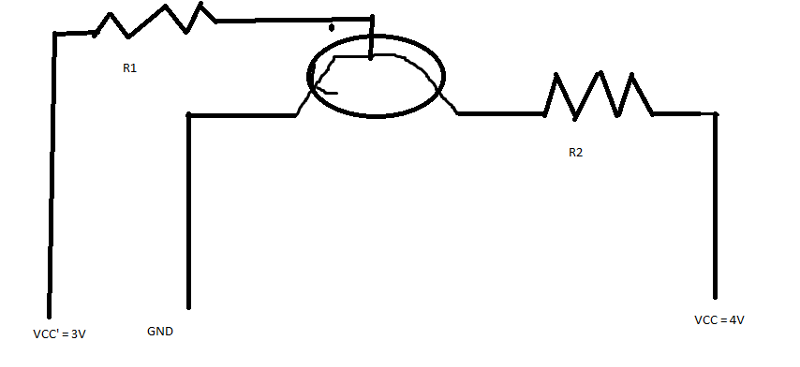why when i increase the value of R1 and voltage drop  in R2 gets bigger?

well im having this trouble for several days.

where am i wrong0
I have a circuit with a transistor and a light emmiting diode:

My source voltage is 11.6 volts

My transistor is in series with 2 resistors and the light emmiting diode.

The resistors values are 1000 Ohm each.

The base is connected to a 0.7 volt supply.

Voltage drop in resistors is 2.7 volts each.

The voltage drop in the light emmiting diode is 2.7 volts

Why is there a 3.4 V voltage drop between emmiter and collector?Shouldnt it be 0 and the voltage drop in the resistors be (11.6-2.7)/2 volts ?

What am i missing?
1
Hello

Need help with a formulas to calculate:
1. (Cell DM23) Most recent past 6-week run rate of 52 week historical data that excludes zeros and high- low numbers (seasonality/Standard Deviation > X).
2.  (Cell DN24) Return data in Range \$BM\$22:\$DL\$3171 in column DN depending on current week written in Cell DM16

(see attached file)
Automate-Run-Rate.xlsx
0

# Math / Science

9K

Solutions

6K

Contributors

The Math / Science topic primarily includes discussions of mathematics, physics, statistics and economic analysis, but also biology, chemistry and other sciences.

Top Experts In
Math / Science
Monthly